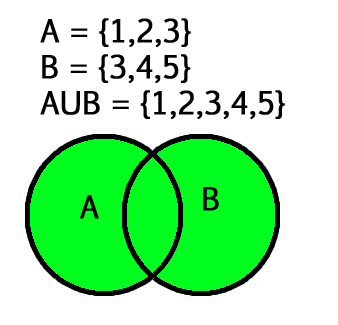Open In App

# Union() function in Python

Python set Union() Method returns a new set that contains all the items from the original set.

The union of two given sets is the set that contains all the elements of both sets. The union of two given sets A and B is a set that consists of all the elements of A and all the elements of B such that no element is repeated.Python Set Union

The symbol for denoting union of sets is ‘U’

## Python Set Union() Method Syntax

The set union() function has the following syntax in Python:

Syntax: set1.union(set2, set3, set4….)

Parameters: zero or more sets

Return: Returns a set, which has the union of all sets(set1, set2, set3…) with set1. It returns a copy of set1 only if no parameter is passed.

## Python set Union() Method Example

Let us see a few examples of the set union() function in Python.

### Python Union() Function on Two Sets

In this example, we will take two Python sets and perform the union() function on them.

## Python3

 `A ``=` `{``2``, ``4``, ``5``, ``6``}``B ``=` `{``4``, ``6``, ``7``, ``8``}` `print``(``"A U B:"``, A.union(B))`

Output:

`A U B: {2, 4, 5, 6, 7, 8}`

### Python Union() Method on Three Sets

In this example, we will take three sets and perform the union() function on them.

## Python3

 `set1 ``=` `{``2``, ``4``, ``5``, ``6``}``set2 ``=` `{``4``, ``6``, ``7``, ``8``}``set3 ``=` `{``7``, ``8``, ``9``, ``10``}` `# union of two sets``print``(``"set1 U set2 U set3 :"``, set1.union(set2).union(set3))` `# union of three sets``print``(``"set1 U set2 U set3 :"``, set1.union(set2, set3))`

Output:

`set1 U set2 U set3 : {2, 4, 5, 6, 7, 8, 9, 10}set1 U set2 U set3 : {2, 4, 5, 6, 7, 8, 9, 10}`

### Python Set Union Using the | Operator

We can use “|” operator to find the union of the sets.

## Python3

 `set1 ``=` `{``2``, ``4``, ``5``, ``6``}``set2 ``=` `{``4``, ``6``, ``7``, ``8``}``set3 ``=` `{``7``, ``8``, ``9``, ``10``}` `# union of two sets``print``(``"set1 U set2 : "``, set1 | set2)` `# union of three sets``print``(``"set1 U set2 U set3 :"``, set1 |set2 | set3)`

Output:

`set1 U set2 :  {2, 4, 5, 6, 7, 8}set1 U set2 U set3 : {2, 4, 5, 6, 7, 8, 9, 10}`

### Python Set Union() Method on String

We can also use set union() method on Python Strings.

## Python3

 `A ``=` `{``'ab'``, ``'ba'``, ``'cd'``, ``'dz'``}``B ``=` `{``'cd'``, ``'ab'``, ``'dd'``, ``'za'``}` `print``(``"A U B:"``, A.union(B))`

Output

```A U B: {'za', 'ab', 'dd', 'dz', 'ba', 'cd'}
```